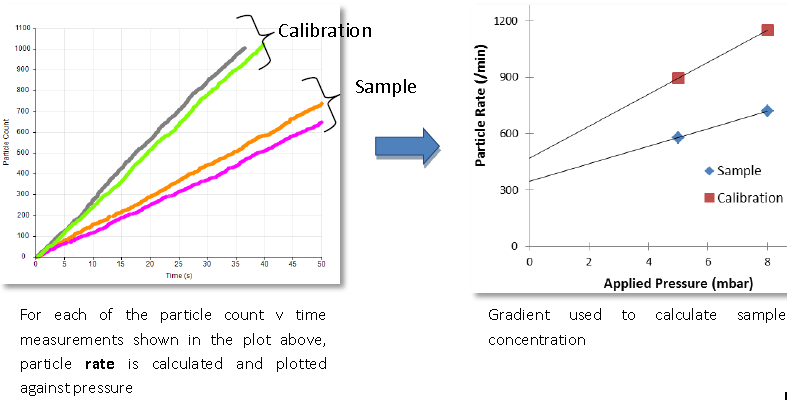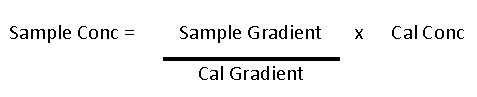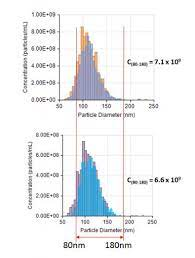Izon Science Support Centre

# How does the instrument measure particle concentration?

The relationship between the particle flux (J) to the particle number concentration (C) and the velocity of particles through the pore (v) is given by:

J = C * v

Particle concentration is determined by measuring particle count rate; the higher the concentration the higher the rate of particles for the same fluid flow.

Fluid flows through the nanopore as a function of applied pressure.

The relationship between the applied pressure and the measured frequency of blockade events is linear for a given sample. Measurement of a reference standard of known size and concentration provides a calibration of the nanopores, against which any other sample can be compared, to obtain an accurate number concentration (number of particles/ml).

Particle concentration is proportional to the change in blockade rate per unit of applied pressure. The figure below shows the rate increase with pressure at 5 and 8 mbar:Particle rate plot can be used to check for system stability. A stable recording gives a linear rate plot. This is crucial as the concentration value is calculated by comparing the gradient of the rate plot of the sample to the calibration measurement:A single pressure concentration measurement calculates a gradient based on the fitted line passing through the origin. This can introduce significant errors, especially with smaller nanopores (NP200 and below) and particles with high surface charge, where a large proportion of particles are driven through the nanopore due to applied voltage.

Accurate concentration values are typically derived at two or more pressures using calibration particles of known size and concentration. Read more about multi-pressure calibration in this article.

Concentration Fraction
TRPS has redefined concentration measurements to determine particle concentrations within a clearly defined particle size range (as displayed in the below image). Other concentration measurement techniques only measure the ‘total’ particle concentration, which will crucially depend on the dynamic size range of the technique used and hence be very subjective.A concentration without stating over which size range it has been measured is not very meaningful.